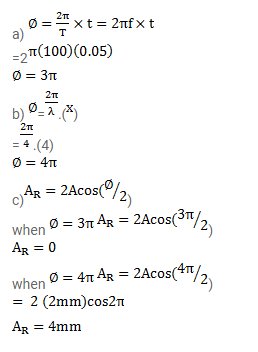# Two waves each having a frequency of

Question:

Two waves each having a frequency of $100 \mathrm{~Hz}$ and a wavelength of $2.0 \mathrm{~cm}$, are travelling in the same direction on a string. What is the phase difference between the waves

(a) If the second wave was produced $0.015 \mathrm{~s}$ later than the first one at the same place

(b) If the two waves were produced at the same instant but the first one was produced at the same instant but the first one was produced at a distance $4.0 \mathrm{~cm}$ behind the second one?

(c) If each of the waves has an amplitude of $2.0 \mathrm{~mm}$, what would be the amplitude of the resultant waves in part (a) and (b)?

Solution: